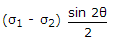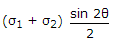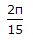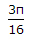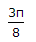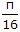# Civil Engineering - Theory of Structures

Exercise : Theory of Structures - Section 4
1.
In case of principal axes of a section
sum of moment of inertia is zero
difference of moment inertia is zero
product of moment of inertia is zero
none of these.
Explanation:
No answer description is available. Let's discuss.

2.
The forces acting normally on the cross section of a bar shown in the given figure introduce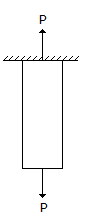compressive stress
tensile stress
shear stress
none of these.
Explanation:
No answer description is available. Let's discuss.

3.
The maximum deflection due to a load W at the free end of a cantilever of length L and having flexural rigidity EI, is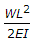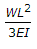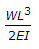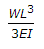Explanation:
No answer description is available. Let's discuss.

4.
If the normal stresses due to longitudinal and transverse loads on a bar are σ1 and σ2 respectively, the tangential component of the stress on an inclined plane through θ°, the longitudinal load is
σ1 sin θ + σ2 cos θ
σ1 sin θ2 + σ2 cos2 θ